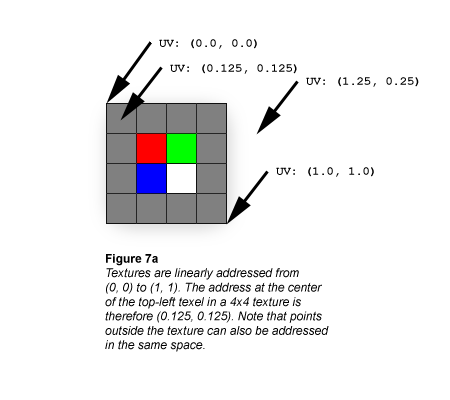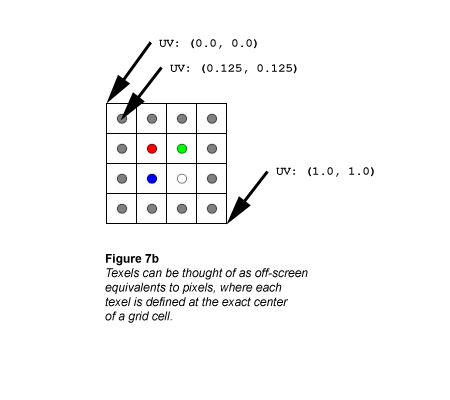# Direct3D 9 (双向纹理筛选)``````UV: (0.5, 0.5)
``````

``````  0.25 * (255, 0, 0)
0.25 * (0, 255, 0)
0.25 * (0, 0, 255)
## + 0.25 * (255, 255, 255)
------------------------
= (128, 128, 128)
``````
``````UV: (0.5, 0.375)
``````

``````  0.5 * (255, 0, 0)
0.5 * (0, 255, 0)
0.0 * (0, 0, 255)
## + 0.0 * (255, 255, 255)
------------------------
= (128, 128, 0)
``````
``````UV: (0.375, 0.375)
``````

``````  1.0 * (255, 0, 0)
0.0 * (0, 255, 0)
0.0 * (0, 0, 255)
## + 0.0 * (255, 255, 255)
------------------------
= (255, 0, 0)
``````